Equations and inequations with square root

Loki123

Junior Member
I have posted a few problems that gave me the same issue. Unfortunately, I haven't been able to understand the reasoning on why they are done the way they are. So I decided to sum up all my problems regarding that issue here in hopes to understand it.
The following picture includes two equations and one inequality. They are not solved completely; rather they all just state a condition revolving the square root.
1st and 3rd problem are equations and they both state that the RHS cannot be negative, that is it must be non-negative. I understand this because LHS is a square root and by definition the square root is always non-negative, that is equal or above 0.
The 2nd problem is giving me a headache. It is an inequality and it is solved by separating the problem into two options; one where x <= 0 and one where x>0. Why is this? My assumption is that although the LHS is always non-negative, the RHS in this case can be negative because it is an inequality. So If RHS is negative and since LHS is always non-negative, the inequality would work because LHS would be always greater than RHS. I tried to present this in a graph. Basically if -x is on the left side of 0, the square root is always greater, however, if -x is on the right side, in some cases the square root would not be greater.
Is this thinking correct? Even if it is, I am still a little bit confused, because why do we have to separate it into two options, why won't solving it like it is just do the job? If we combine x<=0 and x>0, x is basically all numbers.
Thank you,Dr.Peterson

Elite Member
The 2nd problem is giving me a headache. It is an inequality and it is solved by separating the problem into two options; one where x <= 0 and one where x>0. Why is this? My assumption is that although the LHS is always non-negative, the RHS in this case can be negative because it is an inequality. So If RHS is negative and since LHS is always non-negative, the inequality would work because LHS would be always greater than RHS. I tried to present this in a graph. Basically if -x is on the left side of 0, the square root is always greater, however, if -x is on the right side, in some cases the square root would not be greater.
Specifically, the RHS can be negative because it is required to be LESS THAN a positive number, which is true of any negative number. Being less than a positive number does not make a number positive.

I am still a little bit confused, because why do we have to separate it into two options, why won't solving it like it is just do the job? If we combine x<=0 and x>0, x is basically all numbers.
It's misleading to ask "why do we HAVE TO"; there are often multiple ways to solve a given problem. Rather, your question is, "why do they choose to".

Now, I wish you had responded to what was said in the other thread, particularly my (implied) question about what was done next. If you had shown the entire answer, I could refer to that to show the need for two cases, in light of what they did. When you have a question about what someone did, you need to show all of what they did, and not just a little part.

But as I said there, I assume that the next step is to square both sides.

In that case, the reason for making separate cases is that squaring has different effects on positive and negative numbers. Consider this: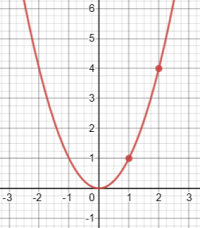For [imath]x>0[/imath], [imath]y = x^2[/imath] is an increasing function, so that given that [imath]2 > 1[/imath], we can conclude that [imath]2^2 > 1^2[/imath].

But if [imath]x<0[/imath], all bets are off: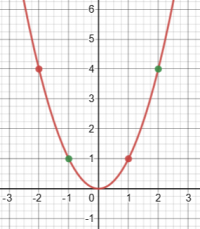Given that [imath]2 > -1[/imath], it is true that [imath]2^2 > (-1)^2[/imath] (the green points); but given that [imath]1 > -2[/imath], it is NOT true that [imath]1^2 > (-2)^2[/imath] (the red points). So if we don't require that the given values are both positive, we can't square them and expect the inequality to be preserved.

So we need to consider separate cases because we are going to be squaring.

So, assuming that the next step is to square and get [imath]x^2-x+1 > x^2[/imath], as I expect, then take an example: for [imath]x = 2[/imath], the original inequality is true, [imath]\sqrt{x^2-x+1}=\sqrt{2^2-2+1}=\sqrt{3}[/imath] while [imath]-x=-2[/imath], and [imath]\sqrt{3}> -2[/imath]; but the squared inequality is not: [imath]\sqrt{x^2-x+1}^2=x^2-x+1=2^2-2+1=3[/imath] while [imath](-x)^2=(-2)^2=4[/imath], and [imath]3 \not{>} 4[/imath].

So you can't square an inequality without imposing a restriction.

•Loki123

Dr.Peterson

Elite Member
Could you explain why they used different conditions than me?
You stated the cases, but don't seem to have done different things in each (which suggests that you don't really understand the reasoning fully):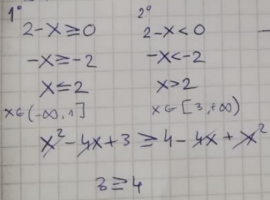It's only in the first case that you can square both sides of the inequality and conclude, as you show, that 3>=4.

In the second case, as I've said, you can't do that, but instead conclude that the inequality is always true, because the LHS is positive and the RHS is negative and therefore necessarily less than the LHS.

It's also worth observing that for this problem, unlike your initial question, the cases they use are not based on the sign of the RHS, but are just the two parts of the domain, each of which happens to imply the sign of the RHS. That aspect of their work is equivalent to what you did. The only difference is that in their first case, they didn't square, but just stated that the inequality is necessarily true.

•Loki123

Loki123

Junior Member
Thank you so much! I think I understand.

Dr.Peterson

Elite Member
Would you mind checking this one out? i got the correct answer, but I am not so sure about how I did it...

First: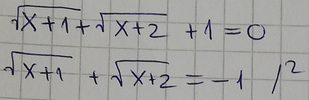You don't need to do the work that follows this, since clearly the LHS can't be negative. But that work isn't wrong, since you know both radicals are defined when [imath]x\ge 1[/imath]. On the other hand, I would have isolated a radical before squaring, to keep things simple. And you could again have stopped when you got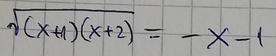since if [imath]x\ge 1[/imath], the RHS has to be negative.

Second: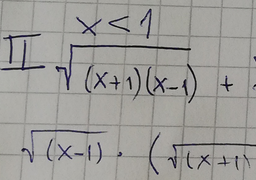Here you have stopped thinking. If you are assuming [imath]x<1[/imath], how can you write the square root of [imath]x-1[/imath], which is negative?

The whole point of using cases is to avoid that! In this case, I would simplify that first term as [imath]\sqrt{(-x-1)(-1+x)}=\sqrt{-x-1}\sqrt{-1+x}[/imath], where the second radical is defined. When is the first radical defined? You'll need to state that, too.

Since the second case is nonsense, you solved the problem by accident. That's not hard to do in a problem like this. But it won't get you a good grade, and doesn't teach you how to think carefully.

Loki123

Junior Member
I would simplify that first term as (−x−1)(−1+x)=−x−1−1+x\sqrt{(-x-1)(-1+x)}=\sqrt{-x-1}\sqrt{-1+x}(−x−1)(−1+x)=−x−1−1+x
I got lost here. Why rewrite it like that and how?

Loki123

Junior Member

First:
View attachment 29313
You don't need to do the work that follows this, since clearly the LHS can't be negative. But that work isn't wrong, since you know both radicals are defined when [imath]x\ge 1[/imath]. On the other hand, I would have isolated a radical before squaring, to keep things simple. And you could again have stopped when you got
View attachment 29314
since if [imath]x\ge 1[/imath], the RHS has to be negative.

Second:
View attachment 29315
Here you have stopped thinking. If you are assuming [imath]x<1[/imath], how can you write the square root of [imath]x-1[/imath], which is negative?

The whole point of using cases is to avoid that! In this case, I would simplify that first term as [imath]\sqrt{(-x-1)(-1+x)}=\sqrt{-x-1}\sqrt{-1+x}[/imath], where the second radical is defined. When is the first radical defined? You'll need to state that, too.

Since the second case is nonsense, you solved the problem by accident. That's not hard to do in a problem like this. But it won't get you a good grade, and doesn't teach you how to think carefully.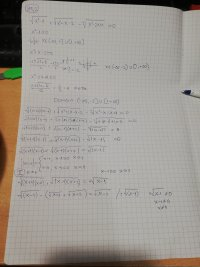Tried a different approach and got that x can't be one... Where did i go wrong??

Loki123

Junior Member

First:
View attachment 29313
You don't need to do the work that follows this, since clearly the LHS can't be negative. But that work isn't wrong, since you know both radicals are defined when [imath]x\ge 1[/imath]. On the other hand, I would have isolated a radical before squaring, to keep things simple. And you could again have stopped when you got
View attachment 29314
since if [imath]x\ge 1[/imath], the RHS has to be negative.

Second:
View attachment 29315
Here you have stopped thinking. If you are assuming [imath]x<1[/imath], how can you write the square root of [imath]x-1[/imath], which is negative?

The whole point of using cases is to avoid that! In this case, I would simplify that first term as [imath]\sqrt{(-x-1)(-1+x)}=\sqrt{-x-1}\sqrt{-1+x}[/imath], where the second radical is defined. When is the first radical defined? You'll need to state that, too.

Since the second case is nonsense, you solved the problem by accident. That's not hard to do in a problem like this. But it won't get you a good grade, and doesn't teach you how to think carefully.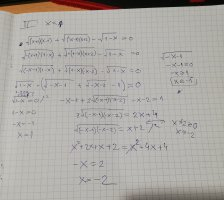I tried to attempt what you described, however, I am pretty sure I did the same thing as before...

Dr.Peterson

Elite Member
Tried a different approach and got that x can't be one... Where did i go wrong??
That doesn't imply that x is not 1; it assumes it in order to divide by 0! The equation you have there is in fact true if x=1.

I'm not convinced that your method is worth pursuing; but if you still want to, you need also to notice the domain of [imath]\sqrt{-x-2}[/imath]

Which of #7 and #10 shows the correct problem?

Loki123

Junior Member
That doesn't imply that x is not 1; it assumes it in order to divide by 0! The equation you have there is in fact true if x=1.

I'm not convinced that your method is worth pursuing; but if you still want to, you need also to notice the domain of [imath]\sqrt{-x-2}[/imath]

Which of #7 and #10 shows the correct problem?
Both. #7 is the entire problem. #10 is a different approach.

Dr.Peterson

Elite Member
Both. #7 is the entire problem. #10 is a different approach.
No, I'm not talking about the work, but about the equation itself. One has a + where the other has a -. I presume the former is a typo, as that equation has no solution.

Is the method you are using your own invention, or is it what you have been taught? I would use an entirely different method.

Loki123

Junior Member
No, I'm not talking about the work, but about the equation itself. One has a + where the other has a -. I presume the former is a typo, as that equation has no solution.

Is the method you are using your own invention, or is it what you have been taught? I would use an entirely different method.
It is -, I don't know how I got that wrong...

Loki123

Junior Member
Specifically, the RHS can be negative because it is required to be LESS THAN a positive number, which is true of any negative number. Being less than a positive number does not make a number positive.

It's misleading to ask "why do we HAVE TO"; there are often multiple ways to solve a given problem. Rather, your question is, "why do they choose to".

Now, I wish you had responded to what was said in the other thread, particularly my (implied) question about what was done next. If you had shown the entire answer, I could refer to that to show the need for two cases, in light of what they did. When you have a question about what someone did, you need to show all of what they did, and not just a little part.

But as I said there, I assume that the next step is to square both sides.

In that case, the reason for making separate cases is that squaring has different effects on positive and negative numbers. Consider this:

View attachment 29167

For [imath]x>0[/imath], [imath]y = x^2[/imath] is an increasing function, so that given that [imath]2 > 1[/imath], we can conclude that [imath]2^2 > 1^2[/imath].

But if [imath]x<0[/imath], all bets are off:

View attachment 29168

Given that [imath]2 > -1[/imath], it is true that [imath]2^2 > (-1)^2[/imath] (the green points); but given that [imath]1 > -2[/imath], it is NOT true that [imath]1^2 > (-2)^2[/imath] (the red points). So if we don't require that the given values are both positive, we can't square them and expect the inequality to be preserved.

So we need to consider separate cases because we are going to be squaring.

So, assuming that the next step is to square and get [imath]x^2-x+1 > x^2[/imath], as I expect, then take an example: for [imath]x = 2[/imath], the original inequality is true, [imath]\sqrt{x^2-x+1}=\sqrt{2^2-2+1}=\sqrt{3}[/imath] while [imath]-x=-2[/imath], and [imath]\sqrt{3}> -2[/imath]; but the squared inequality is not: [imath]\sqrt{x^2-x+1}^2=x^2-x+1=2^2-2+1=3[/imath] while [imath](-x)^2=(-2)^2=4[/imath], and [imath]3 \not{>} 4[/imath].

So you can't square an inequality without imposing a restriction.
hello, sorry for getting this thread active again, however, i am wondering something. In the first post I put three problems and conditions for them. Could you explain to me the reasoning behing the 1st problem and its condition? Because I don't understand why we need that condition. For example if I have sqrtLHS = RHS I am going to write a condition that LHS must be non negative, wouldn't that imply that RHS will also be non negative? Why do I have to write a condition that RHS must be non negative?

Dr.Peterson

Elite Member
hello, sorry for getting this thread active again, however, i am wondering something. In the first post I put three problems and conditions for them. Could you explain to me the reasoning behind the 1st problem and its condition? Because I don't understand why we need that condition. For example if I have sqrtLHS = RHS I am going to write a condition that LHS must be non negative, wouldn't that imply that RHS will also be non negative? Why do I have to write a condition that RHS must be non negative?
You're referring to

I'm not sure I'd call this a "condition"; that, in my mind, would be a restriction on the problem. I'd call it an inference from the problem, based on the fact that we only take the positive square root. The LHS is by definition non-negative; whoever wrote this wanted to remember what this implies about x, to eliminate extraneous solutions due to violation of this fact.

(The fact that the radicand must be non-negative, if that is what you mean by "if I have sqrtLHS = RHS I am going to write a condition that LHS must be non negative", is a separate issue; it is required in order for the entire LHS to exist (as a real number), not for the radical itself (the entire LHS) to be non-negative.)

So, yes, [imath]x<4[/imath] is an implication from the LHS. And you don't have to write it! I myself would usually just find a solution and check against the problem to make sure it isn't extraneous. What you are asking about is just one particular style of work, not something that is required.

If you continue the work, you find that [imath]x=2\pm\sqrt{5}[/imath]; if you check in the equation (which we call plugging each solution in), you find that the positive solution fails because the RHS is negative, while the negative solution works. In the method you are using, you catch the former because it fails the condition you found, so you would never check it. That is the value of your method.

•Loki123

Jomo

Elite Member
Top problem of post #7:

Each term on the left hand side is either 0 or positive.
The right hand side is 0.
Then each term on the left hand side must be 0 (since they are all be added to one another)

Set each term to 0 and find the common answer(s).

•Loki123